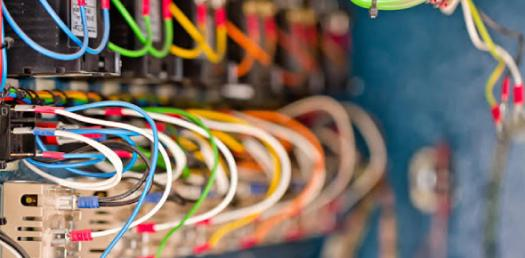# Dgca Module 03 Electrical Fundamentals Question

52 Questions | Total Attempts: 696Settings• 1.
In what equipment is a photon radiated when an electron leaves a hole?.
• A.

Photo-cell.

• B.

LED.

• C.

Photo diode.

• 2.
Elements such as phosphorus with 5 electrons in their outer shell, when combined with pure silicon, is a.
• A.

Isotopic.

• B.

Acceptor.

• C.

Donor.

• 3.
An atom with less than 4 electrons in its outer shell has.
• A.

Low electrical resistance.

• B.

No electrical resistance.

• C.

High electrical resistance.

• 4.
Two inductive coils are placed in close proximity with each other at 90 degrees. The number of flux linkages is.
• A.

0

• B.

Maximum negative.

• C.

Maximum

• 5.
Three inductors 10 mH, 5 mH and 20 mH are connected in parallel. What is the total inductance?.
• A.

Without knowing the coupling factor the total inductance cannot be found.

• B.

2.86mH

• C.

35mH

• 6.
The time constant of an inductor is.
• A.

L/R

• B.

L2 /R.

• C.

LR.

• 7.
If the rate of change of current is halved, mutual inductance will_______
• A.

Stay the same

• B.

Halve

• C.

Double

• 8.
When more than two inductors of different inductance are connected in parallel in a circuit, the total inductance is.
• A.

Equal to the sum of the individual inductance.

• B.

Equal to the inductance of the highest rated inductor.

• C.

Less than the inductance of the lowest rated inductor.

• 9.
The time constant in an inductive circuit is the time required for the current to reach.
• A.

70.7% of maximum value

• B.

63.2% of maximum value

• C.

63.7% of maximum value

• 10.
An induced current in a coil.
• A.

Opposes the EMF producing it.

• B.

Does not affect the EMF producing it.

• C.

Aids the EMF producing it.

• 11.
Capacitance is _________  to plate  surface  area.
• A.

Directly proportional

• B.

Inversely proportional

• C.

Same

• 12.
Which Of  the below  statement  is  true 1. Inductance is more,  More no of flux lines 2. Inductance is less,  Less no of flux lines 3. Inductance is more,   Less no of flux lines
• A.

Both 1 and 3 true

• B.

Both 1 and 2 are true

• C.

Only 1 is true

• 13.
In thermister, if temperature  increases  its resistance ___________
• A.

Increases

• B.

Decreases

• C.

Remain same

• 14.
Unit of power is
• A.

Joule

• B.

Joule/sec

• C.

Joule/min2

• 15.
An inductor is said to be saturated when.
• A.

The current is at a maximum

• B.

The current through it is zero

• C.

It will no longer accept lines of flux.

• 16.
In a DC motor, the stator's magnetic field, due to armature reaction, moves.
• A.

In the same direction of rotation of the armature.

• B.

In the opposite direction of rotation of the armature.

• C.

Into alignment with the GNA.

• 17.
A small air gap between magnetic poles results.
• A.

In a weaker field than a large air gap, for the same magnetising force.

• B.

In a stronger field than a large air gap, for the same magnetising force.

• C.

In the same field as a large air gap, for the same magnetising force.

• 18.
Decreasing the field current in a shunt motor will.
• A.

Decrease speed and increase torque.

• B.

Increase speed and increase torque.

• C.

Increase speed and decrease torque.

• 19.
To calculate generator output you need to know the.
• A.

Armature speed and number of series conductors.

• B.

Armature speed and field strength.

• C.

Armature speed and number of parallel conductors.

• 20.
In a shunt motor, if you reverse both field current and the armature current, the motor will.
• A.

Stop.

• B.

Change direction.

• C.

Continue to run in the same direction.

• 21.
If a generator  speed  is  halved  and  the field strength is doubled, the voltage output will be.
• A.

Unchanged.

• B.

Doubled.

• C.

Halved.

• 22.
How can the  direction of rotation of a DC electric motor be changed?.
• A.

Reverse the electrical connections to either the field or armature windings.

• B.

Rotate the positive brush one commutator segment.

• C.

Interchange the wires which connect the motor to the external power source.

• 23.
The only practical method  of maintaining a constant voltage output from an aircraft generator under varying conditions of speed and load is to vary the.
• A.

Rotates speed at which the armature

• B.

Strength of the magnetic field.

• C.

Number of conductors in the armature.

• 24.
Electric motors are often classified according to the method of connecting the field coils and armature. Aircraft engine starter motors are generally of which type?.
• A.

Compound.

• B.

Shunt (parallel).

• C.

Series.

• 25.
A series wound DC motor, the field has.
• A.

Few turns of thin wire.

• B.

Many turns of thin wire.

• C.

Few turns of thick wire. few turns of thick wire. few turns of thick wire.

Related TopicsBack to top